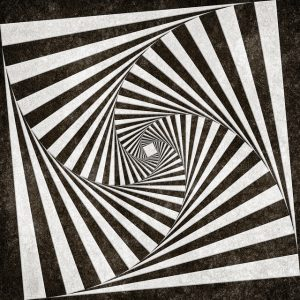Want to share your content on R-bloggers? click here if you have a blog, or here if you don't.Using loops is generally discouraged in R when it is possible to avoid them using vectorized alternatives. Vectorized solution are be both faster to write, read and execute – except sometimes they aren’t and the definition of vectorization isn’t always straightforward.

In any event, solutions using loops can be:

• The fastest to prototype
• The easiest to understand for people coming from other programming language
• Impossible to avoid – the only way to solve a specific problem.

Furthermore, loops are a great tool to play around with in order to gain a deeper understanding of R.

Solutions are available here.

Exercise 1

Write a for loop that iterates over the numbers 1 to 7 and prints the cube of each number using `print()`.

Exercise 2

Write a for loop that iterates over the column names of the inbuilt iris dataset and print each together with the number of characters in the column name in parenthesis. Example output: `Sepal.Length (12)`. Use the following functions `print()`, `paste0()` and `nchar()`.

Exercise 3

Write a while loop that prints out standard random normal numbers (use `rnorm()`) but stops (breaks) if you get a number bigger than 1.

Exercise 4

Using `next` adapt the loop from last exercise so that doesn’t print negative numbers.

Exercise 5

Using a for loop simulate the flip a coin twenty times, keeping track of the individual outcomes (1 = heads, 0 = tails) in a vector that you preallocte.

Exercise 6

Use a nested for loop (a for loop inside a for loop) that produces the following matrix, preallocate the matrix with `NA` values.

```    0     1     2     3     4
1     0     1     2     3
2     1     0     1     2
3     2     1     0     1
4     3     2     1     0
```

Exercise 7

Use a while loop to investigate the number of terms required before the product

1⋅2⋅3⋅4⋅…

reaches above 10 million.

Exercise 8

Use a while loop to simulate one stock price path starting at 100 and random normally distributed percentage jumps with mean 0 and standard deviation of 0.01 each period. How long does it take to reach above 150 or below 50?

Exercise 9

Implement a simple version of Guess the number game using a while loop. The user should guess a number between 1 and 10, you can use `scan()` to get user input. The loop should break if the user guesses 5.

Exercise 10

Implement a multiplication game. A while loop that gives the user two random numbers from 2 to 12 and asks the user to multiply them. Only exit the loop after five correct answers. Try using `as.integer(readline())` instead of `scan()` this time.

If you enjoyed this exercise set you might also enjoy Writing custom functions or Answer probability questions with simulation.

(Image by Nicolas Raymond)